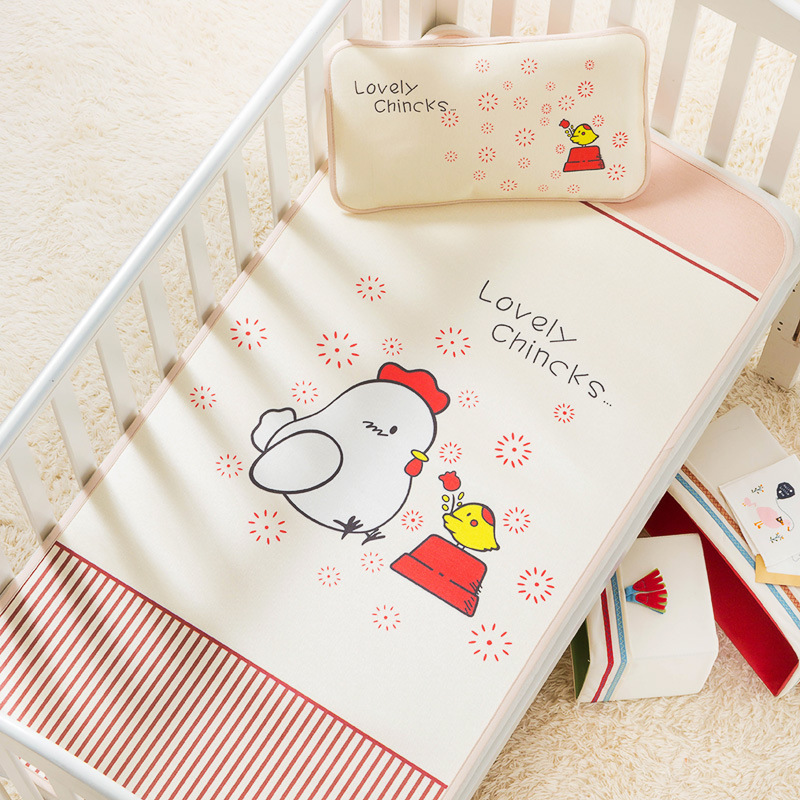1 2 0 * 6 0 c m B a b y c o t m a t i c e w i r e w a s h a b l e s u m m e r n e w b o r n c h i l d b a b y K i n d e r g a r t e n N A P P i l l o w M a t K i t Z - 5 0 1 0

Updated Wednesday 09-Oct-20191 2 0 * 6 0 c m B a b y c o t m a t i c e w i r e w a s h a b l e s u m m e r n e w b o r n c h i l d b a b y K i n d e r g a r t e n N A P P i l l o w M a t K i t Z - 5 0 1 0 0

THB 407.62

More details at ezbuy.co.th